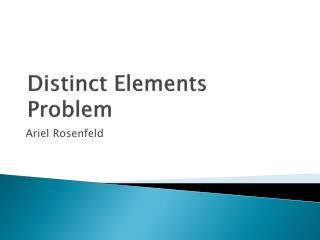DownloadDownload PresentationDistinct Elements Problem

# Distinct Elements Problem

Télécharger la présentation## Distinct Elements Problem

- - - - - - - - - - - - - - - - - - - - - - - - - - - E N D - - - - - - - - - - - - - - - - - - - - - - - - - - -
##### Presentation Transcript

1. Distinct Elements Problem Ariel Rosenfeld

2. Definition • Input: a stream of m integers i1, i2, ..., im. (over 1,…,n) • Output: the number of distinct elements in the stream. • Example – count the distinct number of IP addresses you encounter.

3. Solutions • Bit vector of size n (mark 1 when encountered) • Keeping all m integers and naively answer. • Sort and count O(min{n,mlogm})

4. Why to approximate? • a determinitic exact algorithm is impossible using o(n) bits. • A deterministic approximation algorithm for this problem providing a (1 ± 1/1000)-approximation using o(n) bits is impossible.

5. Idealized Streaming Algorithm (ISA) • Pick random hash function h : [n] → [0, 1] • Calculate z = mini∈stream h(i) • Output 1/z − 1

6. Why is that good? • Same ints gets same hash value. • We will show that the output is a good approximation.

7. Problem • This is idealized for 2 reasons: 1.We don’t have perfect precision. 2. We need n bits at least to remember the randomness associated with every i. Lets ignore it for now…

8. Some notation • S = {j1,…jt} (unique elements in the stream) • h(j1), ..., h(jt) = X1, ..., Xt are independent variables from Unif[0, 1] • Z = min{Xi}

9. In our use 1 P=1 0 1 F(x) 1 0 1

10. . • . (HW) We get a bounded variance.

11. Averaging!

12. q increases -> better approximation Chebyshev

13. What about the hash? • We want a function that doesn't need n bits or more to represent. • So we will use k-wise independent hash functions (H) each can be represented using a small number of bits (log|H|). • In lecture.

14. An example - Set q > k a prime power, and deﬁne Hpoly,kto be the set of all degree ≤ (k − 1) polynomials in Fq[x]. • Hpoly,kis a k-wise independent family. • Size: qk • Needs: k log q bits.